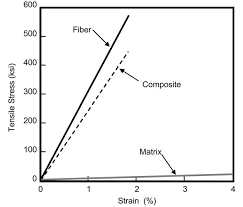## How to Calculate and Solve for Ratio of Load of Fibre to Matrix | Composites

The ratio of load of fibre to matrix is illustrated by the image below.To compute for ratio of load of fibre to matrix, four essential parameters are needed and these parameters are Elastic Modulus of the Fibre (Ef), Elastic Modulus of the Matrix (Em), Volume Fraction of the Fibre (Vf) and Volume Fraction of the Matrix (Vm).

The formula for calculating ratio of load of fibre to matrix:

F(f/m) = EfVf/EmVm

Where:

F(f/m) = Ratio of Load of Fibre to Matrix
Ef = Elastic Modulus of the Fibre
Em = Elastic Modulus of the Matrix
Vf = Volume Fraction of the Fibre
Vm = Volume Fraction of the Matrix

Let’s solve an example;
Find the ratio of load of fibre to matix when the elastic modulus of the fibre is 14, the elastic modulus of the matrix is 10, the volume fraction of the fibre is 6 and the volume fraction of the matrix is 2.

This implies that;

Ef = Elastic Modulus of the Fibre = 14
Em = Elastic Modulus of the Matrix = 10
Vf = Volume Fraction of the Fibre = 6
Vm = Volume Fraction of the Matrix = 2

F(f/m) = EfVf/EmVm
F(f/m) = (14)(6)/(10)(2)
F(f/m) = (84)/(20)
F(f/m) = 4.2

Therefore, the ratio of load of fibre to matrix is 4.2.

Calculating the Elastic Modulus of the Fibre when the Ratio of Load of Fibre to Matrix, the Elastic Modulus of the Matrix, the Volume Fraction of the Fibre and the Volume Fraction of the Matrix is Given.

Ef = F(f/m) x EmVm / Vf

Where:

Ef = Elastic Modulus of the Fibre
F(f/m) = Ratio of Load of Fibre to Matrix
Em = Elastic Modulus of the Matrix
Vf = Volume Fraction of the Fibre
Vm = Volume Fraction of the Matrix

Let’s solve an example;
Find the elastic modulus of the fibre when the ratio of load of fibre to matrix is 20, the elastic modulus of the matrix is 4, the volume fraction of the matrix is 2 and the volume fraction of the fibre is 12.

This implies that;

F(f/m) = Ratio of Load of Fibre to Matrix = 20
Em = Elastic Modulus of the Matrix = 4
Vf = Volume Fraction of the Fibre = 12
Vm = Volume Fraction of the Matrix = 2

Ef = F(f/m) x EmVm / Vf
Ef = 20 x (4)(2) / 12
Ef = 20 x 8 / 12
Ef = 160 / 12
Ef = 13.33

Therefore, the elastic modulus of the fibre is 13.33